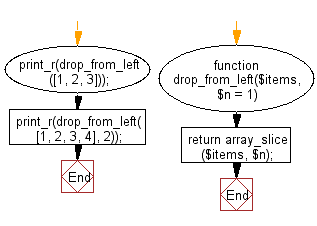﻿ PHP Exercise: Create a new array with n elements removed from the left - w3resource

# PHP Exercises: Create a new array with n elements removed from the left

## PHP: Exercise-80 with Solution

Write a PHP program to create a new array with n elements removed from the left.

Sample Solution: -

PHP Code:

``````<?php
function drop_from_left(\$items, \$n = 1)
{
return array_slice(\$items, \$n);
}
print_r(drop_from_left([1, 2, 3]));
print_r(drop_from_left([1, 2, 3, 4], 2));

?>
```
```

Sample Output:

```Array
(
 => 2
 => 3
)
Array
(
 => 3
 => 4
)```

Flowchart:PHP Code Editor:

Have another way to solve this solution? Contribute your code (and comments) through Disqus.

What is the difficulty level of this exercise?

Test your Programming skills with w3resource's quiz.

﻿

## PHP: Tips of the Day

Mutates the original array to filter out the values specified

Example:

```<?php
function tips_pull(&\$items, ...\$params)
{
\$items = array_values(array_diff(\$items, \$params));
return \$items;
}

\$items = ['x', 'y', 'z', 'x', 'y', 'z'];
print_r(tips_pull(\$items, 'y', 'z'));
?>
```

Output:

```Array
(
 => x
 => x
)
```